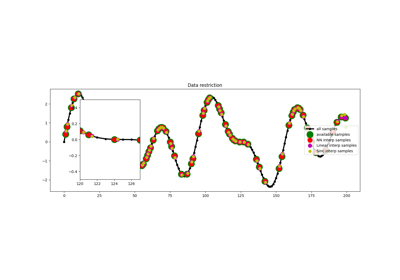# pylops.signalprocessing.Interp¶

pylops.signalprocessing.Interp(M, iava, dims=None, dir=0, kind='linear', dtype='float64')[source]

Interpolation operator.

Apply interpolation along direction dir from regularly sampled input vector into fractionary positions iava using one of the following algorithms:

• Nearest neighbour interpolation is a thin wrapper around pylops.Restriction at np.round(iava) locations.
• Linear interpolation extracts values from input vector at locations np.floor(iava) and np.floor(iava)+1 and linearly combines them in forward mode, places weighted versions of the interpolated values at locations np.floor(iava) and np.floor(iava)+1 in an otherwise zero vector in adjoint mode.
• Sinc interpolation performs sinc interpolation at locations iava. Note that this is the most accurate method but it has higher computational cost as it involves multiplying the input data by a matrix of size $$N \times M$$.

Note

The vector iava should contain unique values. If the same index is repeated twice an error will be raised. This also applies when values beyond the last element of the input array for linear interpolation as those values are forced to be just before this element.

Parameters: M : int Number of samples in model. iava : Floating indices of locations of available samples for interpolation. dims : list, optional Number of samples for each dimension (None if only one dimension is available) dir : int, optional Direction along which restriction is applied. kind : str, optional Kind of interpolation (nearest, linear, and sinc are currently supported) dtype : str, optional Type of elements in input array. op : pylops.LinearOperator Linear intepolation operator iava : Corrected indices of locations of available samples (samples at M-1 or beyond are forced to be at M-1-eps) ValueError If the vector iava contains repeated values. NotImplementedError If kind is not nearest, linear or sinc

pylops.Restriction
Restriction operator

Notes

Linear interpolation of a subset of $$N$$ values at locations iava from an input (or model) vector $$\mathbf{x}$$ of size $$M$$ can be expressed as:

$y_i = (1-w_i) x_{l^{l}_i} + w_i x_{l^{r}_i} \quad \forall i=1,2,\ldots,N$

where $$\mathbf{l^l}=[\lfloor l_1 \rfloor, \lfloor l_2 \rfloor,\ldots, \lfloor l_N \rfloor]$$ and $$\mathbf{l^r}=[\lfloor l_1 \rfloor +1, \lfloor l_2 \rfloor +1,\ldots, \lfloor l_N \rfloor +1]$$ are vectors containing the indeces of the original array at which samples are taken, and $$\mathbf{w}=[l_1 - \lfloor l_1 \rfloor, l_2 - \lfloor l_2 \rfloor, ..., l_N - \lfloor l_N \rfloor]$$ are the linear interpolation weights. This operator can be implemented by simply summing two pylops.Restriction operators which are weighted using pylops.basicoperators.Diagonal operators.

Sinc interpolation of a subset of $$N$$ values at locations iava from an input (or model) vector $$\mathbf{x}$$ of size $$M$$ can be expressed as:

$\DeclareMathOperator{\sinc}{sinc} y_i = \sum_{j=0}^{M} x_j \sinc(i-j) \quad \forall i=1,2,\ldots,N$

This operator can be implemented using the pylops.MatrixMult operator with a matrix containing the values of the sinc function at all $$i,j$$ possible combinations.

## Examples using pylops.signalprocessing.Interp¶Restriction and Interpolation